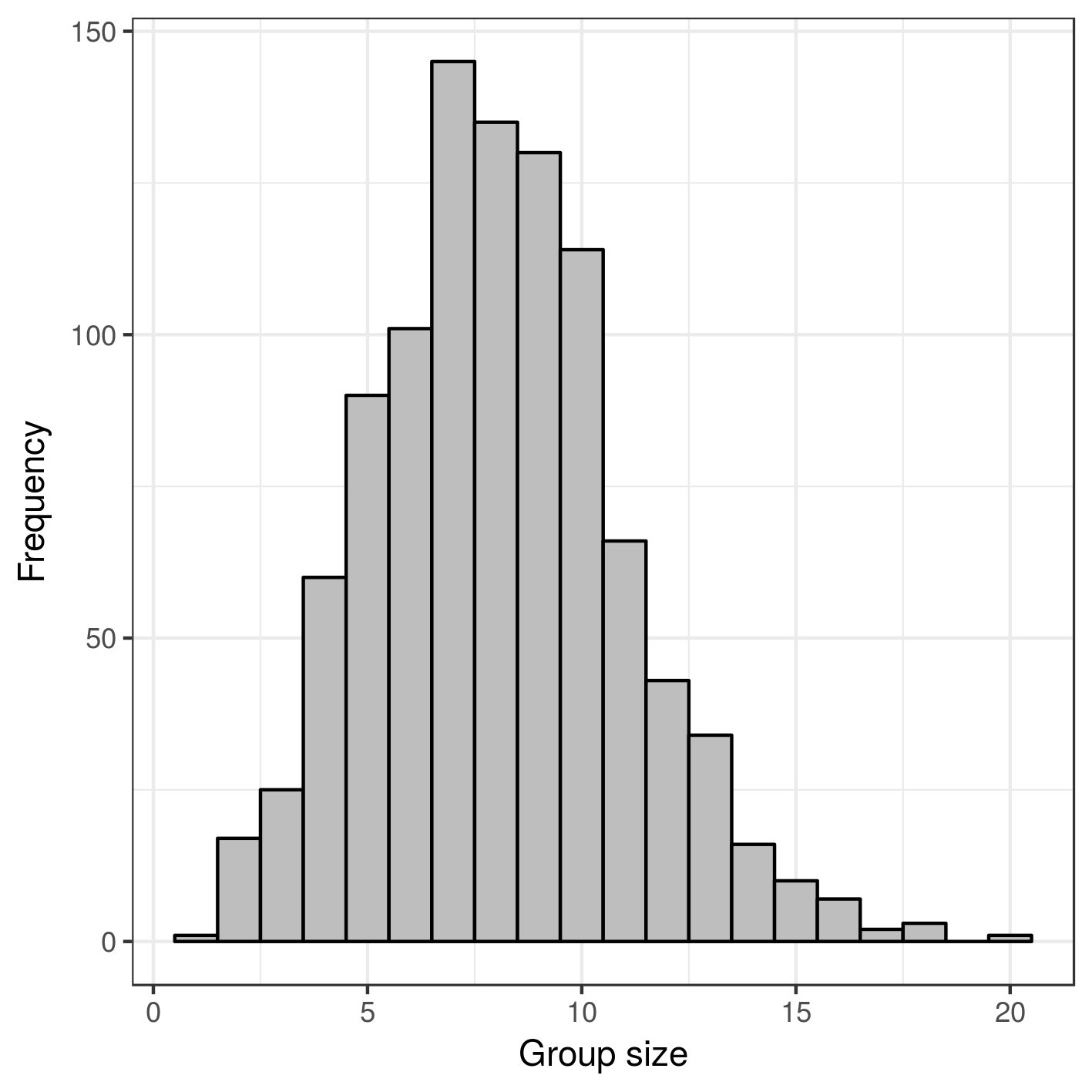The R package grpSLOPE was used to perform the simulation studies presented in (the submitted journal version of) Brzyski, D., Gossmann, A., Su, W., & Bogdan, M. (2016). Group SLOPE — adaptive selection of groups of predictors. Here we show the resulting figures, and link to the R code that can be used to reproduce these simulation studies.

## Figure 2

### Figure 2 a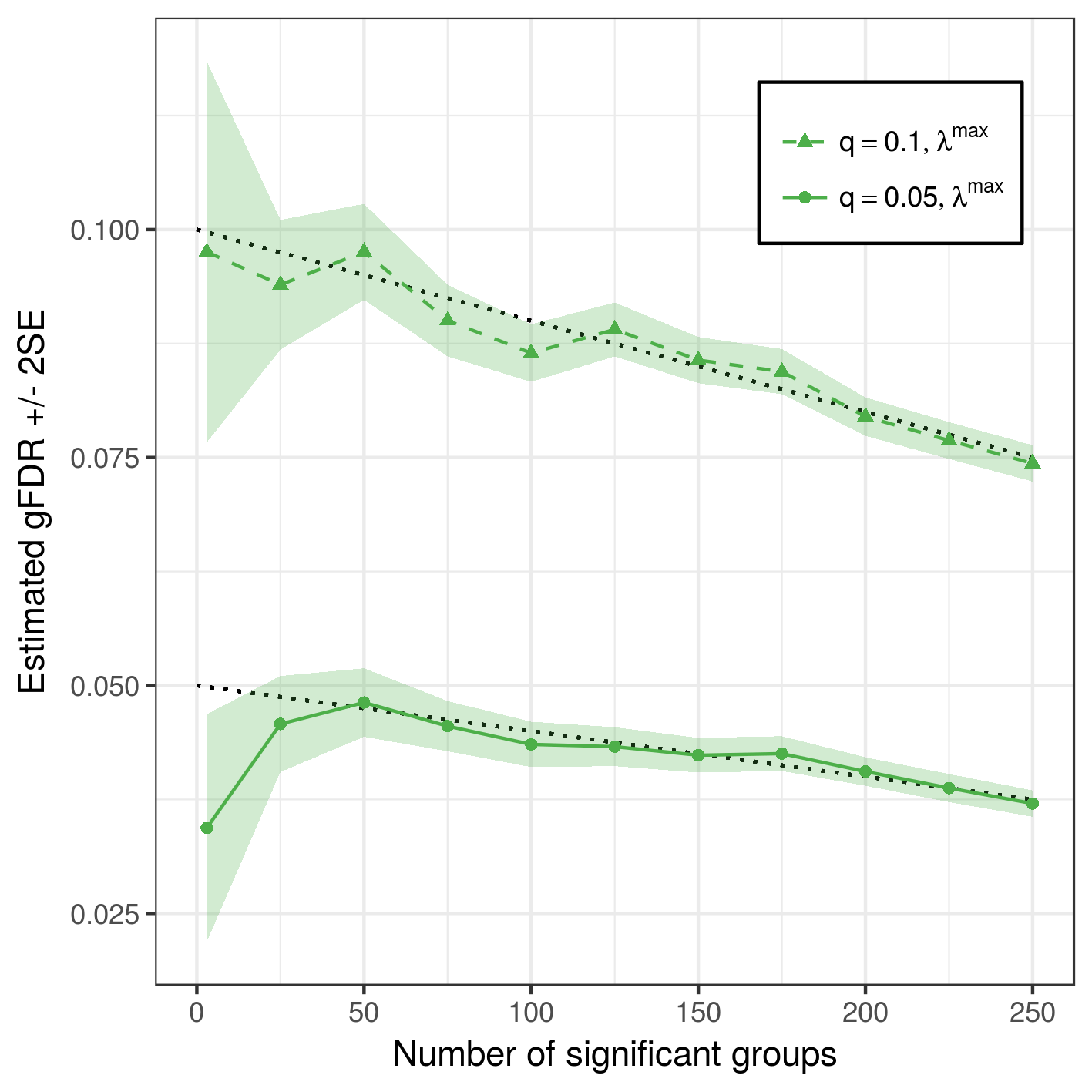### Figure 2 b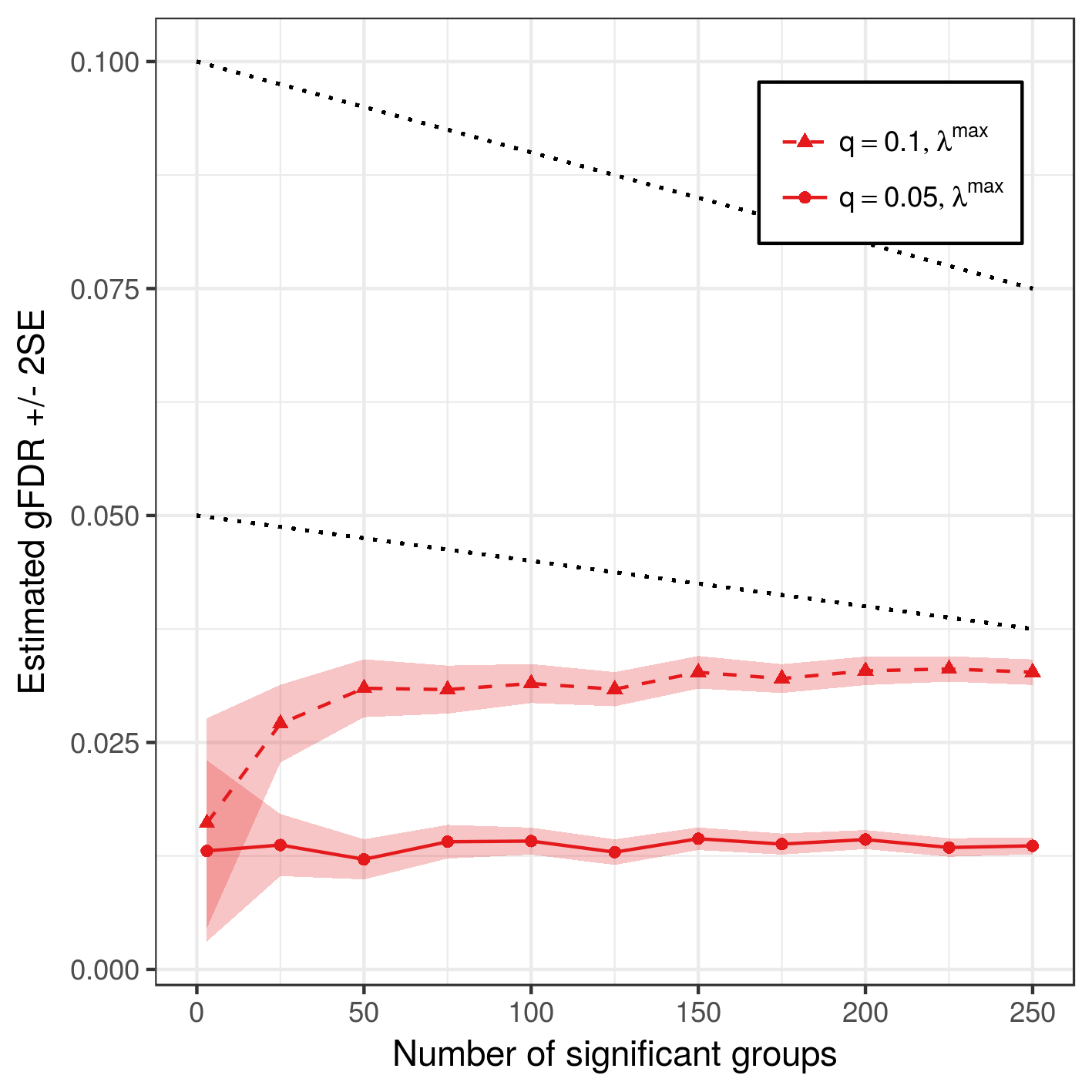### Figure 2 c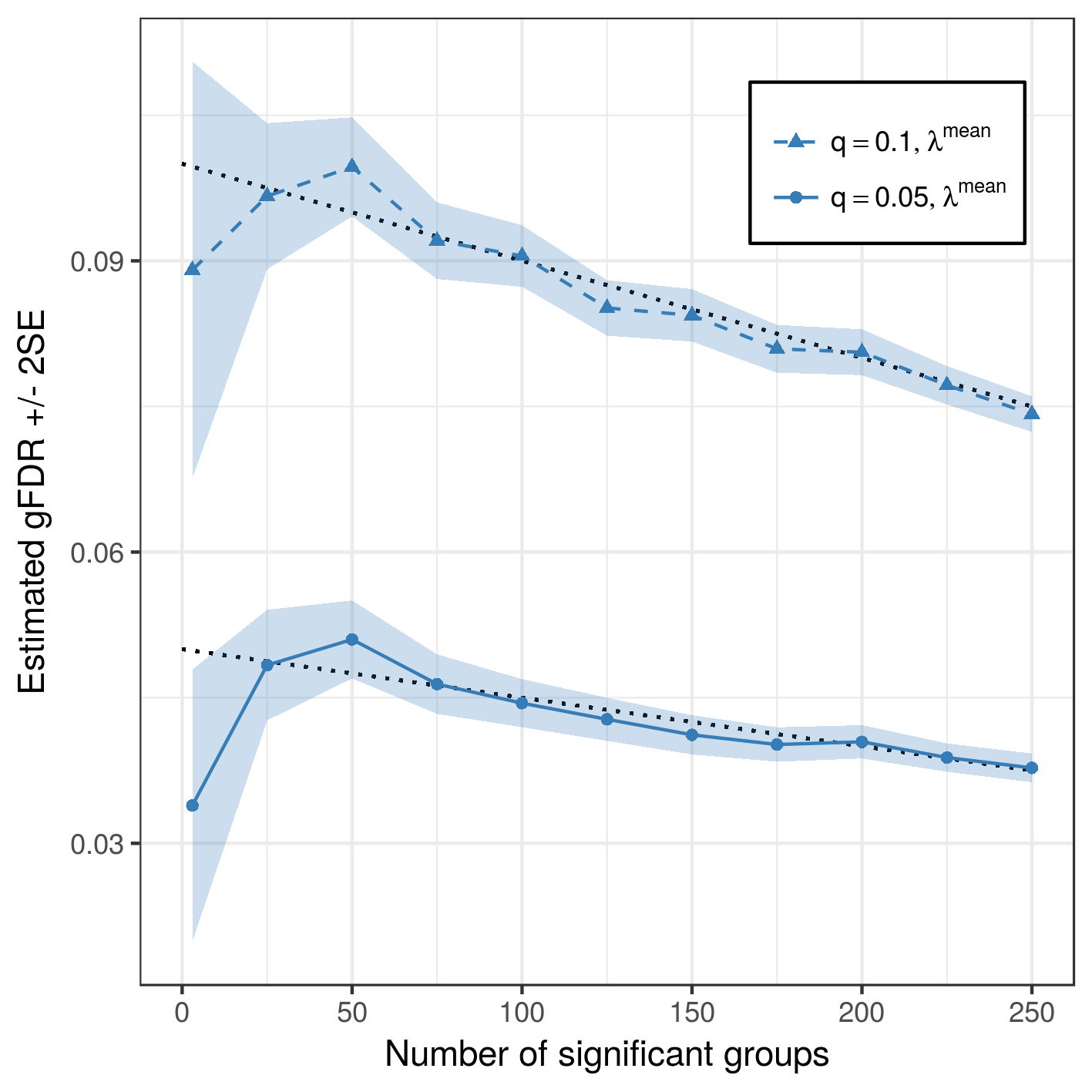### Figure 2 d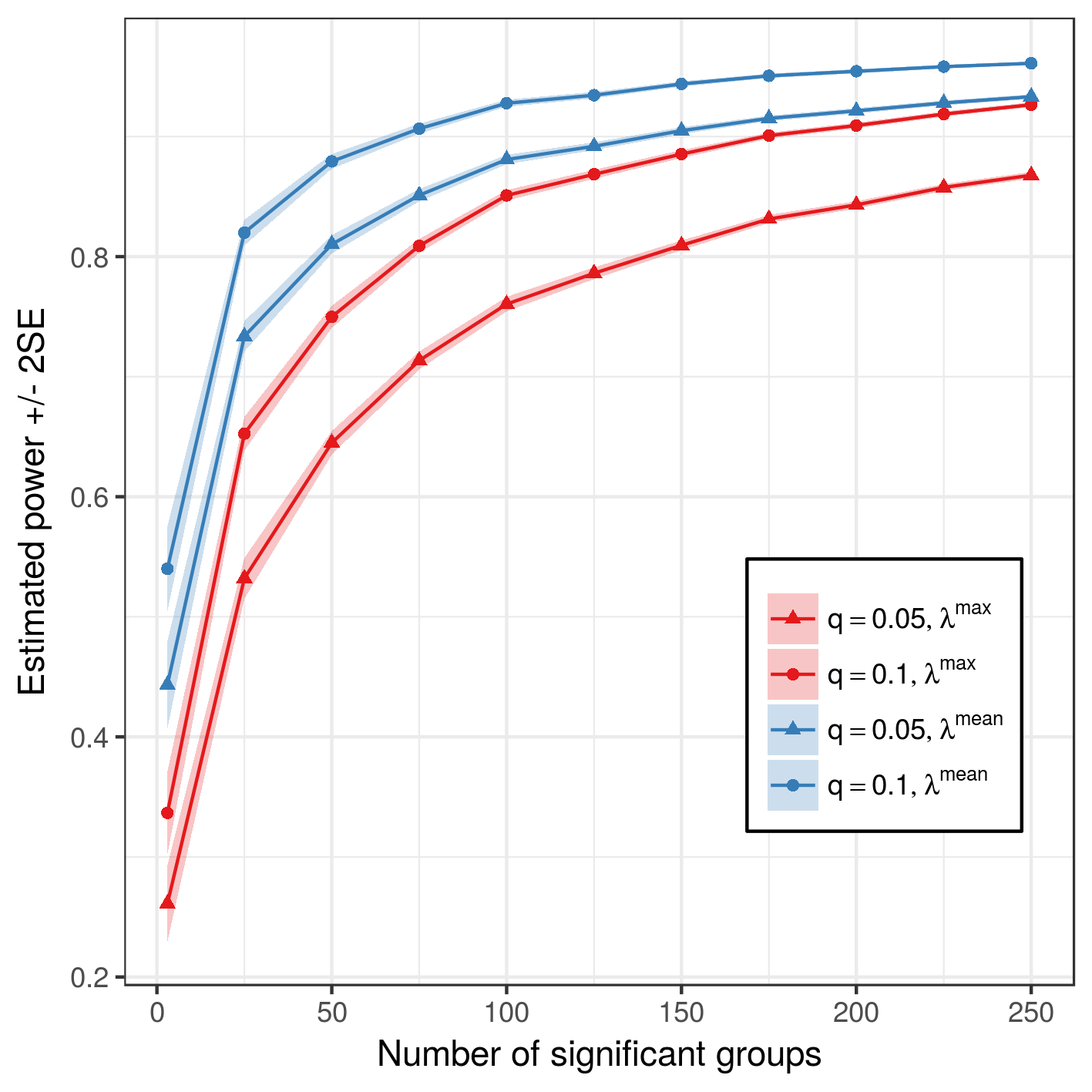### Figure 2 e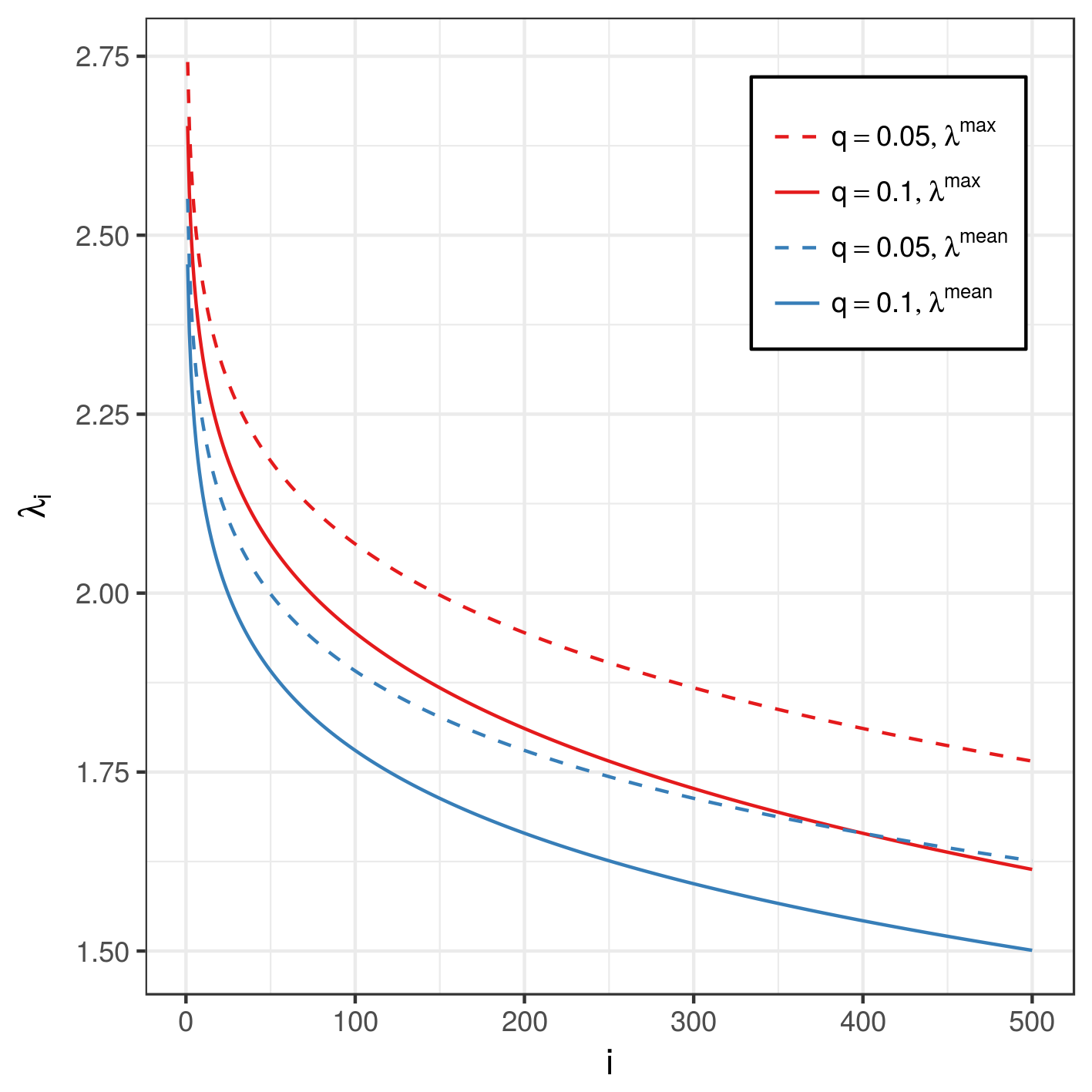## Figure 3 a-c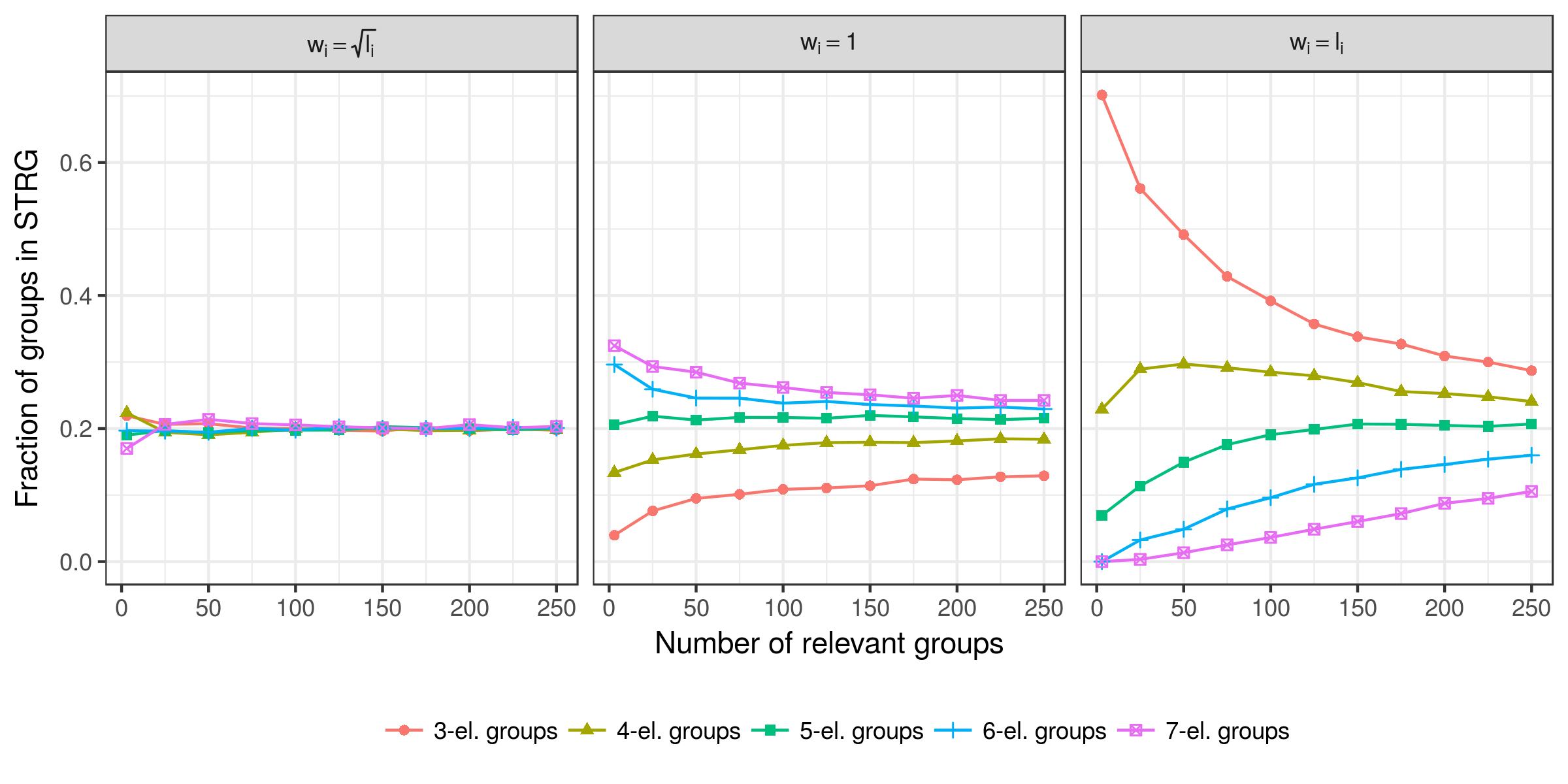## Figure 4

### Figure 4 a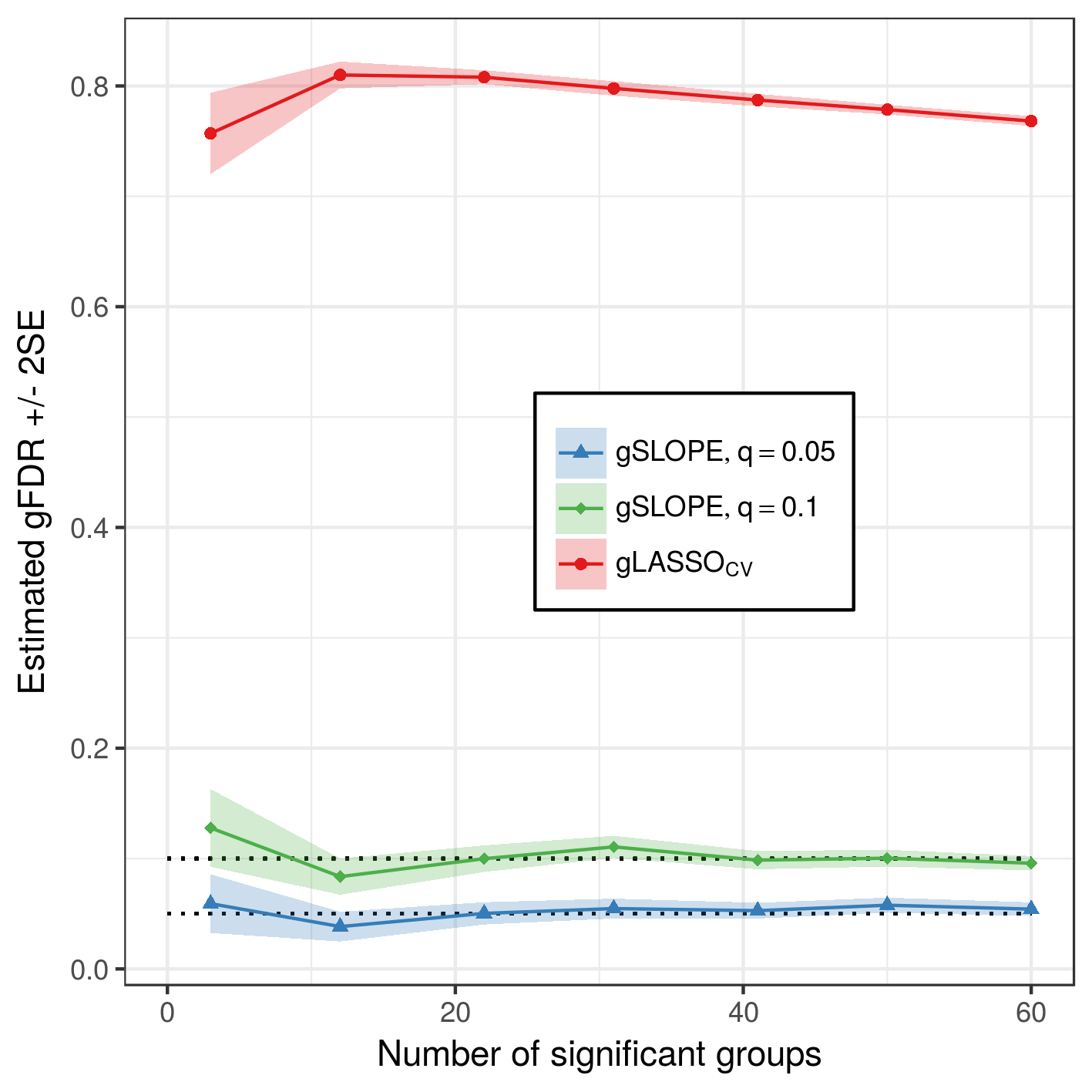### Figure 4 b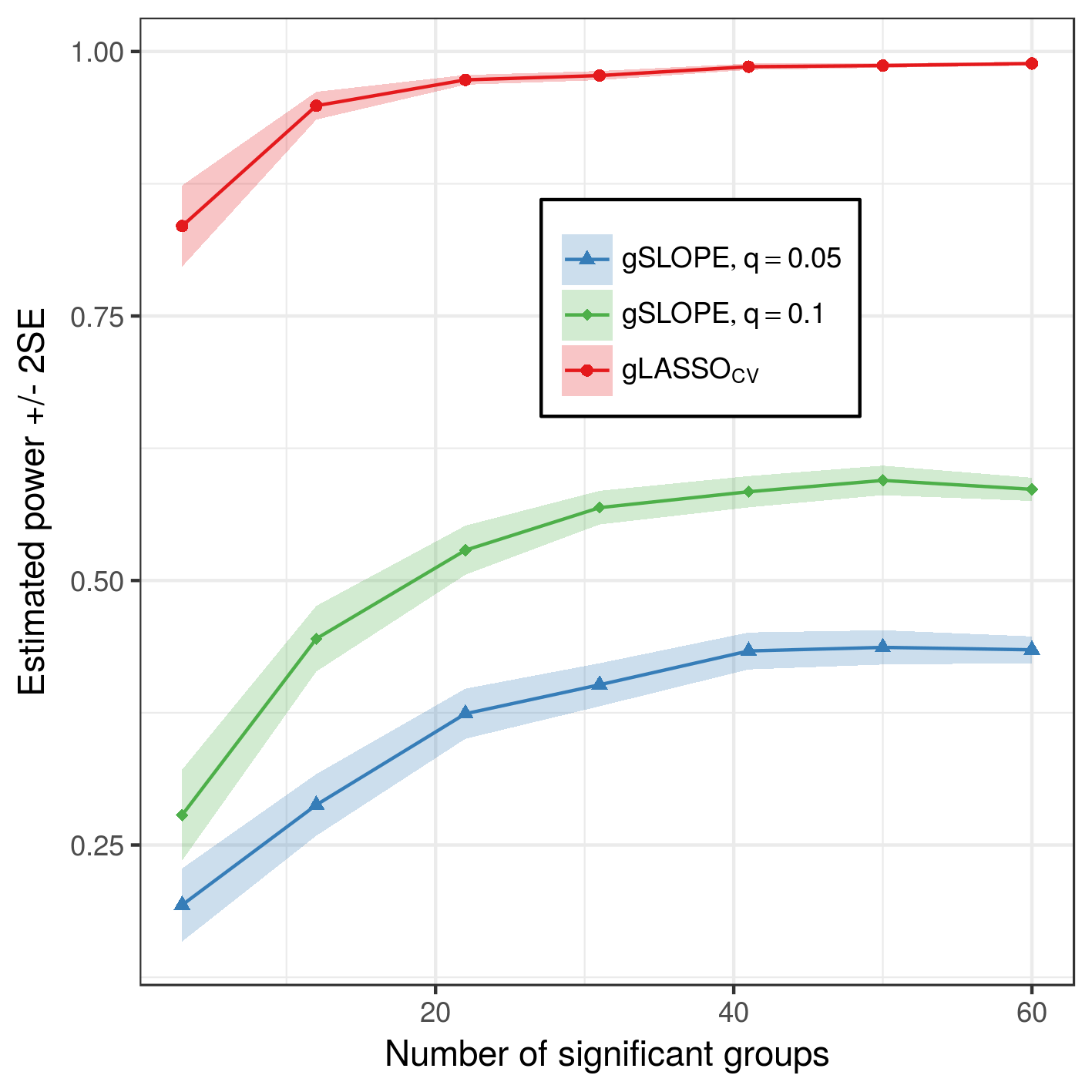### Figure 4 c